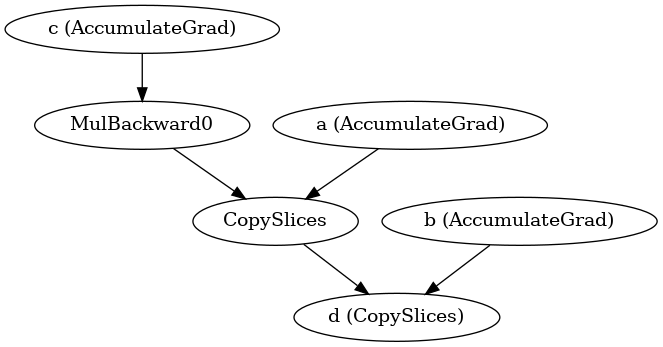# Assign values in a tensor to 0 to stop building computation graph

Hi, I’m having CUDA out of memory issue now, and I’m thinking to reduce some useless variables in the computation graph to save memory.

Particularly, I have a tensor `sim_score` which is calculated in the forward pass by some previous encoders. e.g., `sim_score = tensor([[1, 2, 3, 4], [5, 6, 7, 8]])`. I also have a mask tensor, e.g., `mask = tensor([[0, 1, 0, 0], [1, 1, 1, 0]])`. I will do something like `output = torch.sum(sim_score * mask, dim=1)`. As you can see, 1, 3, 4, 8 in `sim_score` are useless in future computation. However, multiplying the mask tensor seems to keep all values in the computation graph. I wonder is there a way to don’t keep those useless values in the graph to save some gpu memory?

I’ve tried to directly assign the values in `sim_score` to 0 like `sim_score[~mask] = 0`, but I got the error: RuntimeError: one of the variables needed for gradient computation has been modified by an inplace operation.

Many thanks!

The autograd graph if per-tensor not per-element, so you cannot mask within a tensor to free the computational graph.

For much the same reason assigning values will not cut the graph but instead insert a new node CopySlice:

``````a = torch.randn(5, requires_grad=True)
d = c * 2
d[1, 1:-1] = a
d[2, 1:-1] = b
``````

will give an autograd graph that looks like this:If you were to draw intermediate graphs, we would see that while the tensor d stays the same, the autograd node directly attached to it moves from MulBackward0 to the CopySlices with the assignments.

Best regards

Thomas

@tom Hi Thomas, thanks for your reply! I have a follow up question, what if I have:

``````a = torch.randn((4, 7), requires_grad=True)
c[1, 1:3] = a[1, 1:3]
c[2, 3:4] = a[2, 3:4]
# instead of operating on a, we use c for future computation
``````

And imagine `a` is calculated by some previous layers. I guess some values in `a` won’t receive gradient in backward pass. But can this save some gpu memory for me or not at all (compared to using `a * mask` for future computation)?

Best
Yujian

No, it cannot, because

1. the tensors will still be there,
2. the building of the graph (what consumes the gpu memory) happens before the masking, so it would not help,
3. the autograd does not know which bits you don’t need because autograd only works with tensor-level information.

So you could only save something if you had separate tensors and could avoid using some of them and let them go out of scope.

Best regards

Thomas

1 Like